• 条件概率密度的有监督参数估计过程。方法有最大似然估计和贝叶斯参数估计法。 最大似然估计 假设参数为确定值，根据似然度最大进行最优估计。 给定数据 D 1 , D 2 . . . D c D_1,D_2...D_c D 1 ​ , D 2 ​ ...
有监督参数估计是指已知分类器结构或函数形式，从训练样本中估计参数。
本文主要介绍贝叶斯决策（详见贝叶斯决策的过程）条件概率密度的有监督参数估计过程。方法有最大似然估计和贝叶斯参数估计法。
最大似然估计
假设参数为确定值，根据似然度最大进行最优估计。
给定数据

D

1

,

D

2

.

.

.

D

c

D_1,D_2...D_c

表示不同类别的样本。假设每类样本独立同分布（i.i.d. 万年不变的假设），用

D

i

D_i

来估计

θ

i

θ_i

，即对每个类求一个判别函数，用该类的样本来估计判别函数的参数。注意区分特征空间和参数空间。参数估计的任务是得到

p

(

x

∣

w

i

)

p(x|w_i)

的形式，是在参数空间进行的。不妨设特征空间为d维，参数空间p维。 为了估计参数，需要如下几个步骤：
求似然（Likelihood）

p

(

D

∣

θ

)

=

∏

k

=

1

n

p

(

x

k

∣

θ

)

p(D|θ) =\prod_{k=1}^{n}p(x_k|θ)

注意，上面这个式子针对的已经是具体的类别

w

i

w_i

了，不要问

w

w

参数去哪了。另外，这里的n代表样本数目，要和前面的类别数目c区分开。这个式子很好理解，即出现我们当前观测到的样本概率，求使它最大化的参数即可。最大化似然

max

⁡

θ

p

(

D

∣

θ

)

→

▽

θ

p

(

D

∣

θ

)

=

0

\max_θp(D|θ)→▽_θp(D|θ)=0

这个梯度是在p维参数空间求解，即

▽

θ

p

=

[

∂

∂

θ

1

.

.

.

.

.

.

∂

∂

θ

p

]

▽_θp= \begin{bmatrix} \frac{\partial}{\partialθ_1}\\ ...\\ ...\\ \frac{\partial}{\partialθ_p} \end{bmatrix}

求解梯度。可求解析解或梯度下降。（常用Log-Likelihood，易求解）当先验

P

(

θ

)

P(\theta)

都相等时等同于最大后验概率（MAP）决策。
高斯密度最大似然估计
以贝叶斯决策过程里给出的高斯密度假设为例，对它进行最大似然参数估计。首先假设

σ

\sigma

已知，对

μ

\mu

进行估计。
单点情况：对于所有样本：估计值即为观测样本均值。
再来看

μ

\mu

和

σ

\sigma

都未知的情况。设数据服从一维高斯分布，

θ

1

=

μ

\theta_1=\mu

，

θ

2

=

σ

2

\theta_2=\sigma^2

:令梯度等于0可求得：

μ

^

=

1

n

∑

k

=

1

n

x

k

\hat{μ}=\frac{1}{n}\sum_{k=1}^nx_k

σ

^

2

=

1

n

∑

k

=

1

n

(

x

k

−

μ

^

)

2

\hat{σ}^2=\frac1{n}\sum_{k=1}^n(x_k-\hat{μ})^2

多维情况，

θ

2

=

Σ

\theta_2=\Sigma

：

μ

^

=

1

n

∑

k

=

1

n

x

k

\hat{μ}=\frac{1}{n}\sum_{k=1}^nx_k

Σ

^

=

1

n

∑

k

=

1

n

(

x

k

−

μ

^

)

(

x

k

−

μ

^

)

T

\hat{\Sigma}=\frac1{n}\sum_{k=1}^n(x_k-\hat{μ})(x_k-\hat{μ})^T

估计结果类似无偏估计。
贝叶斯参数估计
参数被视为随机变量，估计其后验分布
我们先来简化一下贝叶斯决策的条件概率密度形式。考虑训练样本对分类决策的影响，后验概率可写作：首先由于先验概率一般可以事先得到，因此通常不考虑样本对它的影响。其次，我们使用的是有监督学习，训练样本自然都会分到各自所属的类中。基于这两点可简化公式，得到公式一：由此我们需处理的其实是c个独立的问题，那么条件概率密度可简写成c个

P

(

x

∣

D

)

P(x|D)

，分别对它们进行估计。
下面引出参数分布估计的过程。假定参数形式已知，即已知

p

(

x

∣

θ

)

p(x|θ)

，为求

p

(

x

∣

D

)

p(x|D)

：

p

(

x

∣

D

)

=

∫

p

(

x

,

θ

∣

D

)

d

θ

=

∫

p

(

x

∣

θ

,

D

)

p

(

θ

∣

D

)

d

θ

由于测试样本x（观测样本）和训练样本D的选取是独立的，因此可写成公式二：

p

(

x

∣

D

)

=

∫

p

(

x

∣

θ

)

p

(

θ

∣

D

)

d

θ

样本独立性是《模式分类第二版》里对这步变换做出的解释。对这一部分说一下我的理解。按书里说的x与D相互独立，那p(x|D)其实直接就可以简写成p(x)，且

p

(

θ

)

p(\theta)

也假定已知（后面会说），直接

p

(

x

)

=

∫

p

(

x

∣

θ

)

p

(

θ

)

d

θ

不就能求了，为什么非要对条件概率密度引入D呢？
其实这样做的目的就是为了强行引入

p

(

θ

∣

D

)

p(\theta|D)

。别忘了

p

(

x

∣

D

)

p(x|D)

实际上是

p

(

x

∣

ω

,

D

)

p(x|\omega,D)

，来自公式一。回顾一下公式一引入D的原因，是尽可能地利用已有的全部信息来估计后验概率

p

(

ω

∣

x

)

p(\omega|x)

，对

p

(

x

∣

D

)

p(x|D)

也是这样。即便训练样本对观测值x没有影响，但我们希望再引入一个受样本影响的reproducing density

p

(

θ

∣

D

)

p(\theta|D)

，让它影响类条件概率的分布。其实相当于重新构造了一个先验，并希望

p

(

θ

∣

D

)

p(\theta|D)

在

θ

\theta

的真实值附近有显著的尖峰（sharp）。通常可以用这个sharp逼近的

θ

^

\hat\theta

来替代真实值，有

p

(

x

∣

D

)

≈

p

(

x

∣

θ

^

)

p(x|D) ≈ p(x|\hat\theta)

。如果估计值的置信度不高（用高斯分布来说即方差较大，sharp不明显。后面会说），也可以按

p

(

θ

∣

D

)

p(\theta|D)

对

θ

\theta

进行采样，带入

p

(

x

∣

θ

)

p(x|\theta)

求平均：总结一下，公式一和公式二是贝叶斯决策和参数估计的两个核心部分。尤其是公式二，我们希望把

p

(

x

∣

D

)

p(x|D)

和

p

(

θ

∣

D

)

p(θ|D)

联系起来，那么已有的训练样本就能通过

p

(

θ

∣

D

)

p(θ|D)

对

p

(

x

∣

D

)

p(x|D)

施加影响。至此我们已经把有监督学习问题（原始分类问题）转换成了一个无监督的概率密度预测问题（估计

p

(

θ

∣

D

)

p(θ|D)

）。
高斯密度贝叶斯估计
对高斯密度假设进行贝叶斯参数估计。
考虑一维情况。

p

(

x

∣

μ

)

∼

N

(

μ

，

σ

2

)

p(x|\mu)\sim N(μ，σ^2)

，假设

σ

2

σ^2

已知，为了预测

p

(

μ

∣

D

)

p(μ|D)

，写成：

p

(

μ

∣

D

)

=

p

(

D

∣

μ

)

p

(

μ

)

∫

p

(

D

∣

μ

)

p

(

μ

)

d

μ

p(μ|D)=\frac{p(D|μ)p(μ)}{\int{p(D|μ)p(μ)dμ}}

由于

p

(

D

∣

μ

)

=

∏

k

=

1

n

p

(

x

k

∣

μ

)

p(D|\mu)=\prod_{k=1}^np(x_k|μ)

，则

p

(

μ

∣

D

)

=

α

∏

k

=

1

n

p

(

x

k

∣

μ

)

p

(

μ

)

p(μ|D)=\alpha\prod_{k=1}^np(x_k|μ)p(μ)

α

\alpha

是原式分母，作为常数项。
假设

p

(

μ

)

∼

N

(

μ

0

，

σ

0

2

)

p(μ)\sim N(μ_0，σ_0^2)

，

μ

0

\mu_0

和

σ

0

2

\sigma_0^2

已知。可以把

μ

0

\mu_0

看作对

μ

\mu

的先验估计，

σ

0

2

\sigma_0^2

看作估计的不确定程度。做正态分布假设只是为了简化后面的数学运算。这一步的重点在于在参数估计过程中我们是已知参数先验概率密度

p

(

μ

)

p(\mu)

的。
公式展开：与μ无关的因子都被归入

α

\alpha

中。可见

p

(

μ

∣

D

)

p(μ|D)

仍符合高斯分布，对照标准形式

p

(

μ

∣

D

)

=

1

2

π

σ

n

e

x

p

(

−

1

2

(

μ

−

μ

n

)

2

σ

n

2

)

p(μ|D)=\frac{1}{\sqrt{2\pi}σ_n}exp(-\frac{1}{2}\frac{(\mu-μ_n)^2}{σ_n^2})

可得到目前为止，已经把先验知识

p

(

μ

)

p(\mu)

和训练样本信息

μ

^

n

\hat\mu_n

结合在一起，估计出了后验概率

p

(

μ

∣

D

)

p(\mu|D)

。把结果直观地写在一起：在这个结果中，

μ

n

\mu_n

表示在观测到n个样本后，对参数

μ

\mu

真实值的最好估计，

σ

n

2

\sigma_n^2

则代表这个估计的不确定性（前面对先验假设也是这么解释的，理解一下高斯分布对参数估计的理论意义）。

σ

n

2

\sigma_n^2

随着n的增大而减小，即增加训练样本后，对

μ

\mu

真实估计的置信度将逐渐提高，呈现一个sharp。这样的过程称为贝叶斯学习过程。
将

p

(

μ

∣

D

)

p(\mu|D)

代入

p

(

x

∣

D

)

=

∫

p

(

x

∣

μ

)

p

(

μ

∣

D

)

d

μ

p(x|D)=\int{p(x|μ)p(μ|D)dμ}

得出

p

(

x

∣

D

)

∼

N

(

μ

n

，

σ

2

+

σ

n

2

)

p(x|D)\sim{N(μ_n，σ^2+σ_n^2)}

。因此，根据已知的

p

(

x

∣

μ

)

∼

N

(

μ

，

σ

2

)

p(x|μ)\sim{N(μ，σ^2)}

，只要用

μ

n

μ_n

替换μ，

σ

2

+

σ

n

2

σ^2+σ_n^2

替换

σ

2

σ^2

即可完成参数估计。
我们观察到，当n趋于无穷时，贝叶斯参数估计与最大似然效果相同。（当然在实际问题当中样本往往是有限的，这里只是形式化地理解）
总结一下贝叶斯估计的一般过程：最大似然和贝叶斯估计的比较
在上面的例子中，用贝叶斯参数估计与ML分别对条件概率密度

p

(

x

∣

ω

)

p(x|\omega)

进行估计，得到的虽然都是高斯分布形式，但这个过程中做的假设是完全不同的。 ML直接假定

p

(

x

∣

ω

)

p(x|\omega)

符合高斯分布，根据训练样本选取确定的参数

μ

^

\hat\mu

和

σ

^

2

\hat\sigma^2

。而贝叶斯估计方法是通过假设已知

p

(

x

∣

θ

)

p(x|θ)

和

p

(

μ

)

p(\mu)

符合高斯分布，推出

p

(

μ

∣

D

)

p(\mu|D)

符合高斯分布， 进而根据公式二推出

p

(

x

∣

D

)

p(x|D)

符合高斯分布。这个分布的sharp作为估计的均值，随样本数增加而改变，且确信度逐渐升高。
高斯分布的例子相对来说有点抽象，《模式分类》里还给了一个简单的例子，比较好理解，尤其是这幅图：非常有助于理解。贝叶斯估计在样本最大值之外还有一个拖尾，这就是考虑了先验

p

(

θ

)

p(\theta)

的结果，告诉我们在x=10附近，条件概率密度仍可能不为0。（详见书中例1 递归的贝叶斯学习）
总的来说，最大似然估计根据训练样本明确估计出最优参数值，而贝叶斯估计目标是求出参数的分布，类似于“参数为0.5的概率为0.8”。虽然在估计时模糊的结果（即近似正确）往往更有用，但贝叶斯估计计算复杂度较高，可理解性较差，因此最大似然估计应用更广泛。
展开全文最大似然估计 贝叶斯估计 贝叶斯分类
• 条件概率 在原因B发生的条件下，结果A发生的概率: 全概率 假如结果A发生的原因有B1，B2…等多种原因，则全概率公式如下： 先验概率   事情还没有发生，根据以往经验和分析得到的概率，在事情发生之前，得到的...
注：A表示事情的结果，B={B1，B2…}表示事情发生的原因
条件概率 在原因B发生的条件下，结果A发生的概率: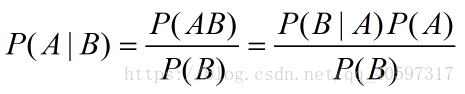全概率 假如结果A发生的原因有B1，B2…等多种原因，则全概率公式如下：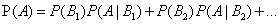先验概率   事情还没有发生，根据以往经验和分析得到的概率，在事情发生之前，得到的事情（结果）发生的概率。比如，一次抛硬币实验，我们认为正面朝上的概率是0.5，这就是一种先验概率，在抛硬币前，我们只有常识。类条件概率 就是已知一个条件下，结果发生的概率。条件概率实际上把一个完整的问题集合S通过特征进行了划分，划分成S1/S2/S3…。类条件概率中的类指的是把造成结果的所有原因一 一进行列举，分别讨论。 贝叶斯公式如下：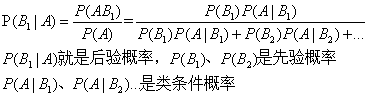后验概率   事情已经发生了，结果的发生的原因有很多，判断结果的发生是由哪个原因引起的概率 贝叶斯公式如下：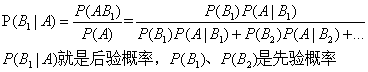如果我们把事件A看做 “结果”，把诸事件B1,B2…看做导致这个结果的可能的“原因”，则可以形象地把全概率公式看做成为“由原因推结果”。　　而贝叶斯公式则恰好相反,其作用于“由结果推原因”：现在有一个“结果”A以发生,在众多可能的“原因”中，到底是哪一个导致了这结果。 举个例子:   桌子上如果有一块肉喝一瓶醋，你如果吃了一块肉，然后你觉得是酸的，那你觉得肉里加了醋的概率有多大？你说：80%可能性加了醋。OK，你已经进行了一次后验概率的猜测。没错，就这么简单。1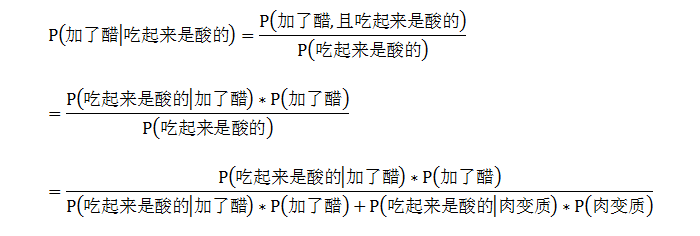进一步思考1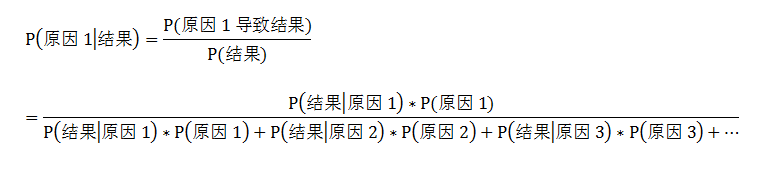那么这个P(原因1导致结果)和P(结果|原因1)之间到底有什么联系呢?让我们举一个图像识别的例子    假如给你一些图片,这些图片中有的图上有动物的角,这些图片占了1/10(即先验概率),且已知在有角的条件下是犀牛的概率是0.8(类条件概率1,注意这个概率互补的概率是有角条件下不是犀牛的概率),已知在无角条件下是犀牛概率的是0.05(类条件概率2),现在拿起一张图,发现是一张犀牛的图,那么这张图上带角的概率有多大(求后验概率)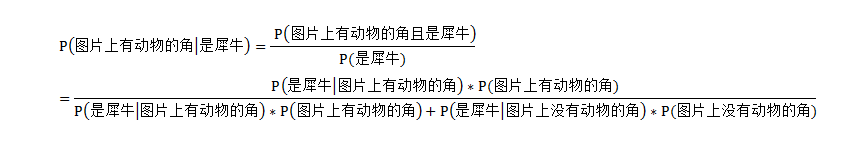由图中公式可知P(图片上由动物的角|是犀牛) = 0.80.1/(0.80.1+0.05*0.9)=0.64    可以看到P(图片上由动物的角且是犀牛)=0.08与P(是犀牛|图片上由动物的角)=0.8之间差别非常大

参考博客https://www.cnblogs.com/yemanxiaozu/p/7680761.html ↩︎ ↩︎

展开全文先验概率 后验概率 贝叶斯公式
• 随机样本的生成和概率密度函数的绘制——模式识别课程作业，希望能有所帮助
• 类条件概率 一个事件发生后另一个事件发生的概率。一般的形式为P(x|y)表示y发生的条件下x发生的概率。 后验概率 事件发生后求的反向条件概率；或者说，基于先验概率求得的反向条件概率。概率形式与条件概率相同。 ....
先验概率
事件发生前的预判概率。可以是基于历史数据的统计，可以由背景常识得出，也可以是人的主观观点给出。一般都是单独事件概率，如P(x),P(y)。
类条件概率
一个事件发生后另一个事件发生的概率。一般的形式为P(x|y)表示y发生的条件下x发生的概率。
后验概率
事件发生后求的反向条件概率；或者说，基于先验概率求得的反向条件概率。概率形式与条件概率相同。
展开全文机器学习
• ## 概率密度函数估计

万次阅读 2017-06-03 17:58:39
最常用的就是比较后验概率的大小，进行类别决策。（也就是基于最小错误率的分类器，还有其他的比如基于最小风险，NP决策等）。  如何理解呢，说一个例子，比如一个班里面的男女比例为2:1，那么也就是说男生占2/3...
        首先来看贝叶斯决策，贝叶斯分类器就是根据如下贝叶斯公式来设计的。最常用的就是比较后验概率的大小，进行类别决策。（也就是基于最小错误率的分类器，还有其他的比如基于最小风险，NP决策等）。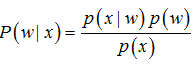如何理解呢，说一个例子，比如一个班里面的男女比例为2:1，那么也就是说男生占2/3，女生占1/3。这个呢就叫做类别的先验概率（类别就是男生、女生），对应公式上的p(w)。接着假设这个班上男生翘课的概率为3/4，女生翘课的概率为1/4，那么这个就叫做类条件概率，也就是类别约束（男生或者女生）下，事件（翘课）的概率，对应上面公式的p(x|w)。需要注意：先验概率满足总和为1的约束，类条件概率不满足总和为1的约束。这也很好理解，因为所有的类别都是固定的，那么一个个体总是属于某个类别中的一个，而类条件概率，比如男生缺课和女生缺课是相互独立的事件，则缺课的概率p(x)=2/3 * 3/4 + 1/3*1/4=7/12。因为不存在约束关系，所以也就不满足总和为1的约束。

上面的例子，现在假设有一个人缺课了，但是不知道是男生还是女生，怎么判断？基于最小错误率的贝叶斯分类器就是利用贝叶斯公式，由先验概率和类条件概率计算后验概率，比较大小，然后进行决策。缺课条件下是男生的概率为（2/3 * 3/4）/ 7/12 = 6/7；缺课条件下是女生的概率为（1/3 * 1/4）/ 7/12 = 1/7。也就是说一个人缺课了，它是男生的概率较大。

但是在实际问题中并不都是这样幸运的，我们能获得的数据可能只有有限数目的样本数据，而先验概率和类条件概率都是未知的。根据仅有的样本数据进行分类时，一种可行的办法是我们需要先对先验概率和类条件概率进行估计，然后再套用贝叶斯分类器。

先验概率的估计较简单，1、每个样本所属的自然状态都是已知的（有监督学习）；2、依靠经验；3、用训练样本中各类出现的频率估计。
类条件概率的估计（非常难），原因包括：1、概率密度函数包含了一个随机变量的全部信息；2、概率密度函数可以是满足下面条件的任何函数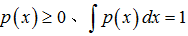；3、在很多情况下，已有的训练样本数总是太少；4、当用于表示特征的向量x的维数较大时，就会产生严重的计算复杂度问题(算法的执行时间，系统资源开销等)。总之要直接估计类条件概率的密度函数很难。

于是就出现了概率密度函数的估计问题，这了讨论的主要是对类条件概率的估计。估计的方法分为两大类：
参数估计(parametric)：
参数估计法，样本所属的类别和类条件概率密度函数形式已知，而表征概率密度函数的某些参数是未知的。要求由已知类别的训练数据样本集，对概率密度的某些参数进行统计估计。如：
最大似然估计
Bayesian估计

非参数估计(non-parametric)：
已知样本所属类别，但未知概率密度函数的形式，要求不用模型，而只利用训练数据本身对概率密度做估计。              Parzen窗方法
Kn近邻估计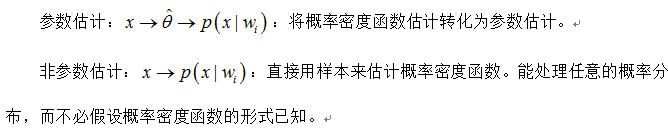展开全文参数估计 非参数估计 模式识别
• 1.条件概率 条件概率反应的是在给定A的条件下B的概率 由条件概率可得 由此还可以推出全概率公式，在全概率公式里，P(A)是所有P(AB_i)的求和，对应概率图表中A的偏概率 2.贝叶斯公式 贝叶斯公式由条件概率...
• 原文来自师兄的博客：...多元正态分布的条件密度 多元正态分布多元正态分布的密度函数如下 ： fx(x1,...xn)=1(2π)k√|Σ|1/2exp(−12(x−μ)TΣ−1(x−μ))f_{x}(x_{1},...x_{n})=\frac{1}{\sqrt{(2\pi)^{多元正太分布
• parzen窗的matlab实现 本文档实现的是用简单的matlab程序实现parzen窗的设计parzen
•matlab
• 笔者在研究室内定位算法的过程中，有一些论文出现了条件均值。比如x∼f(x)x\sim f(x)，那么该变量的均值为 EX=∫+∞−∞xf(x)dx\begin{equation*} EX=\int_{-\infty }^{+\infty }{xf(x)dx} \end{equation*} 现在...条件均值
• 接上一篇文章：最大似估计贝叶斯估计： 参数估计 是...贝叶斯学习是把贝叶斯估计的原理应用于直接从数据对概率密度进行估计开始我们今天的表演一、贝叶斯估计可以将概率密度函数参数估计问题看成是贝叶斯决策问题 ...贝叶斯估计
• 通过Matlab实现了FSO链路的负指数分布、K分布、Gamma-Gamma分布模型的概率密度函数，可以对比分析三种分布的概率密度函数，并可以根据画出不同湍流强度条件下的pdf。
• 总结一下这次作业的知识点~ 1.数据导入 最简便的方法是将文件添加到左侧区域，右键“导入数据”，就可以进入导入数据的页面啦！ 需要注意：导入数据中设定的变量名，输出类型（列矢量、表...）。...matlab
• 什么是先验概率 条件概率 和后验概率? 先验概率就是凭借统计或者常识或者其他手段先天就已经知道的概率,比如我知道冰皮今晚要看片的概率是0.8 条件概率,就是在冰皮要看片的条件下,冰皮Lol的概率是0.9 后验...
• 1. 条件概率密度 2. 条件概率密度求解示例1 3. 条件概率密度求解示例2 4. 条件概率密度求解示例3
• 转载自：... 在统计学中似然函数(Likelihood function)与概率密度函数(Probability density function)都扮演着重要的角色。本文针对的是其在参数估计（Paramet...
• [mu,sigma]=normfit(x); r=normrnd(mu,sigma,1000,1); 根据样本x计算mu和sigma, 使用normrnd生成1000个服从样本概率密度函数的随机数。matlab 数据分析
• 机器学习（二）概率密度估计之非参数估计 2018/2/19 by ChenjingDing 二.非参数估计 2.1直方图估计 直方图估计概率密度函数基本思想： 将数据空间分成许多个子空间，每一个子空间大小为△△△，在每一个子...
• （2） 联合分布律和联合概率密度的区别 离散型的通过数据枚举的方式获得其本身的联合分布律（Joint distribution law） 连续型的本质是需找到ke'y可以代表的概率密度函数   （3）边缘分布 ...
• 在贝叶斯分类（这里有个简介：...但是，在实际应用中，类条件概率密度通常是未知的。那么，当先验概率和类条件概率密度都未知或者其中之一未知的情况下，该如何来进行类别判断呢？其实，只要我们能收集
• 本文地址：http://blog.csdn.net/shanglianlm/article/details/498398331 引言概率密度估计分为参数估计（概率密度函数形式已知，部分或全部参数未知）和非参数估计（概率密度函数也未知）。1-1 一些基本概念1-2 ...函数 模式识别
• 所以我们引入概率密度函数，一目了然看出落在x的某一值附近的概率大小（两方面理解：1.连续不说某一值的概率，而是区间。2.概率的大小是积分，而当积分区域无限小，就可以看成一条直线，即y值）
• 之前的博客中已经提到，贝叶斯决策的基础是概率密度函数的估计，即根据一定的训练样本来估计统计决策中用到的先验概率P(wi)P(w_i)P(wi​)和类条件概率密度p(x∣wi)p(x|w_i)p(x∣wi​)。 概率密度函数的估计分为参数...极大似然估计
• 已知先验分布概率和条件概率，使用贝叶斯公式，求后验分布的概率
• 很多教程中把概率分布和积分是混在一起讲的, 个人觉得分开讲比较合适. 这篇文章就先来讲下概率分布变换和随机采样的部分.概率论基础这里快速回顾下概率论的基础, 这里不会特别深入精确地描述. 需要的朋友可以参考...离散分布的分布函数
• 联合概率密度 P(A^B) 条件概率 从面积比例看出，P(A|B)等于B中A的面积（P(A^B)）除以B的面积（P(B)）。 乘法公式（乘积法则） 假如事件A与B相互独立，那么： 相互独立：表示两个事件互不影响。 互斥：......